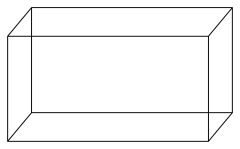# Calculate 7653

The block volume is 900cm3, and the surface is 600cm2. The area of one wall is 60cm2. Calculate the length of edges a, b, c.

a =  15 cm
b =  10 cm
c =  6 cm

### Step-by-step explanation:Did you find an error or inaccuracy? Feel free to write us. Thank you!

Tips for related online calculators
Are you looking for help with calculating roots of a quadratic equation?
Do you have a system of equations and looking for calculator system of linear equations?